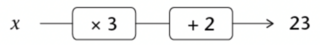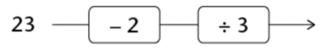Home Practice
For learners and parents For teachers and schools
Textbooks
Full catalogue
Pricing SupportLog in

We think you are located in United States. Is this correct?

# Solving equations using additive and multiplicative inverses

## 9.2 Solving equations using additive and multiplicative inverses

To solve a linear equation, we do the same thing to both sides of the equation to get the variable on its own.

Consider the equation, $$3x + 2 = 23$$.

We can represent this equation in a flow diagram, where $$x$$ represents an unknown number:When you reverse the process in the flow diagram, you start with the output number $$23$$, then subtract $$2$$ and then divide the answer by $$3$$:We can write the steps in this reverse process as follows:

Subtract $$2$$ from both sides of the equation:

\begin{align} 3x + 2 &= 23 \\ 3x + 2 - 2 &= 23 - 2 \\ 3x &= 21 \end{align}

Divide both sides by $$3$$:

\begin{align} 3x &= 21 \\ 3x \div 3 &= 21 \div 3 \\ x &= 7 \end{align}

We say $$x = 7$$ is the solution of $$3x + 2 = 23$$, because $$3 \times 7 + 2 = 23$$.

We say that $$x = 7$$ makes the equation $$3x + 2 = 23$$ true.

The numbers $$+ 2$$ and $$- 2$$ are additive inverses of each other. When we add a number and its additive inverse, we always get $$0$$, so $$2 + ( - 2) = 0$$.

The numbers $$3$$ and $$\frac{1}{3}$$ are multiplicative inverses of each other. When we multiply a number and its multiplicative inverse, we always get $$1$$, so $$3 \times \frac{1}{3} = 1$$.

The additive and multiplicative inverses help us to isolate the unknown value or the input value.

a number that results in the answer $$0$$ when it is added to the original number
multiplicative inverse
a number that results in the answer $$1$$ when it is multiplied by the original number

Also remember:

• The multiplicative property of $$1$$: the product of any number and $$1$$ is that number.
• The additive property of $$0$$: the sum of any number and $$0$$ is that number.

## Worked example 9.1: Using the additive inverse

Solve for $$b$$:

$- 2 = b + 4$

### Get $$b$$ on its own.

To get $$b$$ on its own, we must do the opposite of what has been done to $$b$$. The $$4$$ has been added to $$b$$, so we must subtract $$4$$ from both sides of the equation.

\begin{align} - 2 &= b + 4 \\ 2 - 4 &= b + 4 - 4 \\ 6 &= b \\ \therefore b &= 6 \end{align}

“Doing the same thing to both sides” to get $$b$$ on its own is also called “applying the inverse operation”.

## Worked example 9.2: Using the multiplicative inverse

Solve for $$b$$:

$- 7 = - 2b$

### Get $$b$$ on its own.

We want the coefficient of $$b$$ to be $$1$$. We have $$-2b$$, which means that $$b$$ is being multiplied by $$-2$$. To get $$b$$ on its own with a coefficient of $$1$$, we must do the opposite of what has been done to $$b$$: we must divide by $$-2$$.

### Do the same thing to the other side of the equation.

Whenever we solve equations, we must do the same thing to both sides to make sure that the value of the equation remains the same. So, we must divide both sides of the equation by $$-2$$.

\begin{align} - 7 &= - 2b \\ - 7 \div \left( - 2 \right) &= - 2b \div \left( - 2 \right) \\ \frac{- 7}{- 2} &= \frac{- 2b}{- 2} \\ \frac{7}{2} &= b \end{align}

$$1b$$ means the same thing as $$b$$ (because when you multiply any number by $$1$$, you get the same number). We usually write $$b$$ instead of $$1b$$ because it is simpler, and mathematicians like things to be as simple as possible.

When working with fractions in the equation, you will use the number called a reciprocal. When a fraction is multiplied by its reciprocal, the answer is $$1$$. For example, $$\frac{4}{5} \times \frac{5}{4} = 1$$. We say that $$\frac{4}{5}$$ and $$\frac{5}{4}$$ are reciprocals of each other.

reciprocal
the inverse or opposite of a value; the product of the number and its reciprocal is equal to $$1$$

## Worked example 9.3: Solving equations with fractions

Solve for $$t$$:

$7 = \frac{3}{4}t$

### Get $$t$$ on its own.

To get $$t$$ on its own, we need to get the coefficient of $$t$$ to be $$1$$. So, we need to think about what number to multiply $$\frac{3}{4}$$ by, so that is becomes $$1$$.

To get rid of the denominator, we need to multiply by $$4$$. To get rid of the numerator, we need to divide by $$3$$.

This is the same as multiplying by $$\frac{4}{3}$$. This number is called the reciprocal of $$\frac{3}{4}$$.

### Multiply by the reciprocal.

We must do this on both sides of the equation.

\begin{align} 7 &= \frac{3}{4}t \\ 7 \times \frac{4}{3} &= \frac{3}{4}t \times \frac{4}{3} \\ \frac{28}{3} &= t \end{align}

You could also have done this in two steps. You could multiply both sides by the denominator first, and then divide both sides by the numerator.

temp text

### Multi-step equations with inverses

Remember that to solve a linear equation, we do the same thing to both sides of the equation to get the variable on its own. To do this we must do the opposite of what has been done to that variable, but keep in mind the order of operations.

## Worked example 9.4: Solving two-step linear equations

Solve for $$b$$:

$- 4 = - 2 + 4b$

### Simplify the equation.

The $$2$$ has been subtracted from the side where $$b$$ is, so we must add $$2$$ to both sides of the equation.

\begin{align} - 4 &= - 2 + 4b \\ - 4 + 2 &= - 2 + 4b + 2 \\ - 2 &= 4b \end{align}

### Get the variable on its own.

Now we can focus on getting the coefficient of $$b$$ to be $$1$$. To get $$b$$ on its own with a coefficient of $$1$$, we must do the opposite of what has been done to $$b$$. We must divide both sides of the equation by $$4$$.

\begin{align} - 2 &= 4b \\ - \frac{2}{4} &= \frac{4b}{4} \\ - \frac{1}{2} &= b \\ b &= - \frac{1}{2} \end{align}

You might have tried to solve this equation by first dividing both sides by the coefficient of $$b$$ (which is $$4$$ in this case). This would give you the correct answer, as long as you remembered to divide $$-2$$ by $$4$$. But this method can create fractions that are difficult to work with. (It is also easy to forget to divide $$-2$$ by $$4$$!) It is best to deal with the number first, and only divide by the coefficient of $$b$$ as the very last step.

## Worked example 9.5: Solving two-step linear equations with decimals

Solve for $$m$$:

$2 + \text{0,2}m = - 3$

### Use inverse operations to solve for $$m$$:

\begin{align} 2 + \text{0,2}m &= - 3 \\ 2 + \text{0,2}m - 2 &= - 3 - 2 \\ \text{0,2}m &= - 5 \\ \text{0,2}m \div \text{0,2} &= - 5 \div \text{0,2} \\ \therefore m &= - 25 \end{align}

If you don’t have a calculator, you might find it easier to convert the decimal into a common fraction:

\begin{align} \text{0,2}m &= - 5 \\ \text{0,2}m \div \text{0,2} &= - 5 \div \text{0,2} \\ m &= - 5 \div \frac{2}{10} \\ m &= - 5 \times \frac{10}{2} \\ m &= - \frac{50}{2} \\ m &= - 25 \end{align}

## Worked example 9.6: Solving two-step linear equations with fractions

Solve for $$z$$:

$\frac{4}{5}z - 4 = 1$

### Solve for $$z$$ using inverse operations.

To solve a linear equation, we do the same thing to both sides of the equation to get $$z$$ on its own. The $$4$$ has been subtracted from the $$z$$-term. So, we must add $$4$$ to both sides of the equation.

\begin{align} \frac{4}{5}z - 4 &= 1 \\ \frac{4}{5}z - 4 + 4 &= 1 + 4 \\ \frac{4}{5}z &= 5 \end{align}

### Get $$z$$ on its own.

Now we need to get the coefficient of $$z$$ to be $$1$$. So, we need to think about what number to multiply $$\frac{4}{5}$$ by to get to $$1$$. To get rid of the denominator, we need to multiply by $$5$$. In the step after that, we will divide by $$4$$ to get rid of the numerator.

\begin{align} \frac{4}{5}z &= 5 \\ \frac{4}{5}z \times 5 &= 5 \times 5 \\ 4z &= 25 \\ \frac{4z}{4} &= \frac{25}{4} \\ z &= \frac{25}{4} \end{align}

You could have also multiplied both sides by the reciprocal, $$\frac{5}{4}$$.

\begin{align} \frac{4}{5}z \times \frac{5}{4} &= 5 \times \frac{5}{4} \\ z &= \frac{25}{4} \end{align}

## Worked example 9.7: Using the distributive law to solve equations

Solve for $$b$$:

$- ( - a + 2) = 2$

### Simplify the left hand side of the equation.

The first thing that we do when solving equations is simplify each side of the equation. We can use the distributive law to simplify the left-hand side of this equation. The right-hand side is already simplified, so we will leave it as is for now.

\begin{align} - ( - a + 2) &= 2 \\ - 1 \times ( - a) - 1 \times (2) &= 2 \\ a - 2 &= 2 \end{align}

### Use inverses to solve for $$a$$.

Now we can solve the equation using inverse operations to isolate $$a$$.

\begin{align} a - 2 &= 2 \\ a - 2 + 2 &= 2 + 2 \\ a &= 4 \end{align}

You could have also solved this equation by first dividing both sides by $$-1$$.

temp text